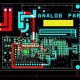# Main Topics for Electrical Engineering.

In this tutorial, we are going to learn about main topics of electrical engineering.

Here below are the main topics of electrical engineering.

• Capacitors – It is a device which store electrical energy in an electric field.
• Inductors – It is a device which stores energy in a magnetic field.
• Transformer – It is a device which transfers electrical energy form one AC circuit to other circuit. It has two types voltage transformer and current transformer.
• Electrical voltage – It is the force from an electrical circuit power source which pushes charged current through a conducting loop, then it us enabling them to do work like as illuminating a light, Electrical voltage measured in volts (V).
• Electrical current – It is a flow of electron, Which is moving through an electrical conductor or space. Electrical current is measured in ampere (V)
• Electrical resistance – It is a use of the opposition to current flow in an electrical circuit. It is measured in ohms which has use symbol omega (Ω).
• Electric power – It is the rate per unit time, there which electrical energy is transferred by an electric circuit and power measured in watt (W) which is equal to  one joule per second.
• Electric charge – This is the physical property of matter which causes it to experience a force when placed in an electromagnetic field.
• Power efficiency – It is the ratio of total output power to input power, which is expressed in percent. Power efficiency is basically measured at full load and nominal input voltage.
• Electrical Motor – It is an electric motor is an electrical machine that converts electrical energy into mechanical energy.
• Electrical Generator – It is a device which converts mechanical energy into electrical energy.
• Inverter – It is a power electronic device which is use for changes direct current (DC) to alternating current (AC).
• UPS – It is full form is uninterruptible power supply (UPS) which is use to allows a  source  to keep running for at least a short time when the primary power source is lost like computer, CNC machine, SMT machine etc.
• SMPS – It is full form is switch mode power supply which is use convert ac into dc.